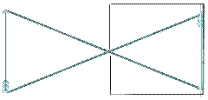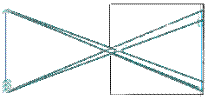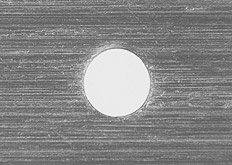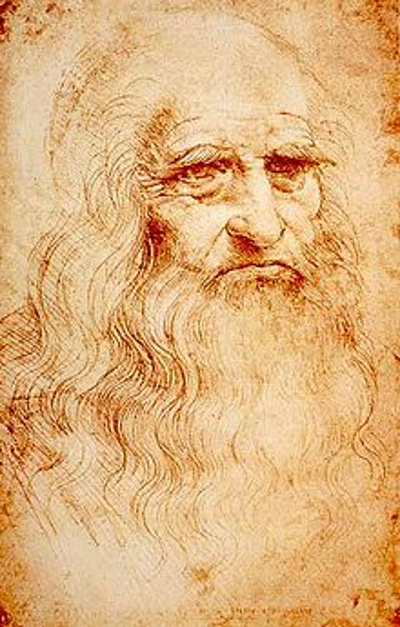Search:

# The pinhole camera

De radio astronomico et geometrico liber (1545).

The German physicist and mathematician Gemma Frisius observed the 1544 solar eclipse in a dark room.

We take a carton!

It should not let pass the light ! A rectangular hole is cut it. We cover the hole with a small metal plate in which is drilled a tiny perfectly round hole. An opaque adhesive tape applied to the metal plate serves as a shutter.

--- The camera is complete! ---

Pinhole

The hole lets pass light rays coming from an object:The image of the object is reversed on the back wall of the box! If the hole is too big, it lets enter the light rays like this:Each point of the object will be projected as a small spot. The image will be blurry! If the hole is too small or irregular, a diffraction phenomenon is observed, which makes the new image imprecise.

The big challenge is therefore to achieve a perfectly round hole with the right size.Perfect $0.2\; mm$ hole (enlarged)

Trial and error!

It is defined for any photo device:

$f$ $=$ $\frac{l}{d}$

with: l = distance from the hole to photographic film d = diameter of the hole

If $f$ is large, the hole is small and the distance to the film is large, so little light reaches the film. To make a good picture, you have a long exposure time (leave the hole open a long time) For example: $l$ $=$ $100mm$,$d$ $=$ $0,4 mm$ → $f$ $=$ $250$ The ideal exposure time dramatically increases (not proportionally) with f! See the beautiful czech →       Pinhole Page with →       a stupendous procedure to build a "Dirkon" camera without a lens !!

A Nobel Prize!

Enters John William Strutt (Lord Rayleigh). This is the chemist and physicist who found why the sky is blue!Rayleigh determined the ideal diameter (in $mm$) of the hole:

$d$ $=$ $\sqrt{l\cdot\lambda}$

with: $l$ = distance from the hole to photographic film $\lambda$ = wavelength of the light which passes through the hole (in $mm$) p. eg for yellow light ($\lambda$ $=$ $0,00055\;mm$) and a focal distance of $10\;cm$ , the ideal diameter of the hole is: $d$ $=$ $0,45\; mm$

Leonardo da Vinci!Evil tongues say that the great scientist and Italian artist Leonardo da Vinci used a dark room to prepare his drawings!